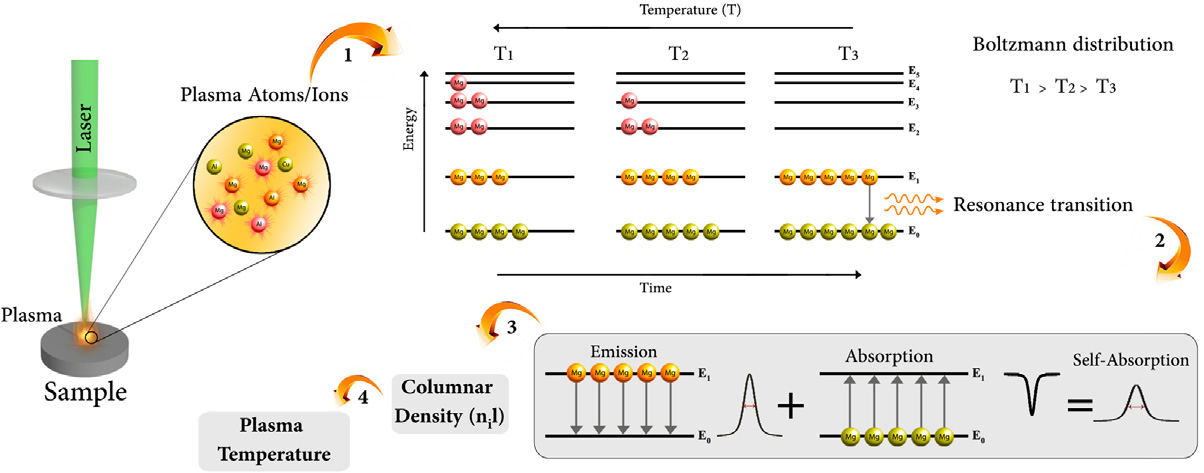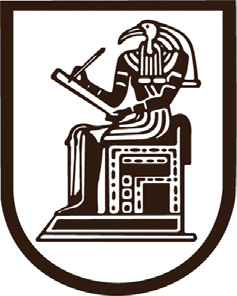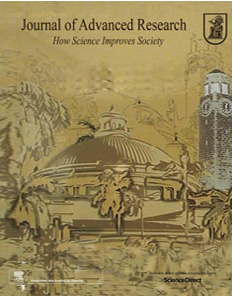Contents lists available at ScienceDirect
Original article
Determination of excitation temperature in laser-induced plasmas using
columnar density Saha-Boltzmann plot
Ali Saﬁa, S. Hassan Tavassolia,, Gabriele Cristoforettib, Stefano Legnaiolic, Vincenzo Palleschic,
Fatemeh Rezaeid, Elisabetta Tognonib
a Laser and Plasma Research Institute, Shahid Beheshti University, G. C., Evin, Tehran, Iran
b National Institute of Optics of the National Research Council (INO-CNR), Via G. Moruzzi 1, Pisa, Italy
c Applied and Laser Spectroscopy Laboratory, Institute of Chemistry of Organometallic Compounds, Research Area of National Research Council, Via G. Moruzzi, 1, Pisa, Italy
d Department of Physics, K. N. Toosi University of Technology, 15875-4416 Tehran, Iran
h i g h l i g h t s
g r a p h i c a l
a b s t r a c t
 Characterization of LIP by the
Columnar Density Saha-Boltzmann
(CD-SB) plot.
 Use of strongly self-absorbed lines to
calculate the plasma temperature.
 Temporal evolution of the plasma
temperature by CD-SB plot.
 CD-SB plot as a promising method to
obtain plasma temperature at later
times.
 CD-SB plot does not require the
calibration of the detection system.
a r t i c l e
i n f o
a b s t r a c t
Article history:
In exploiting the analytical capabilities of plasma-based spectroscopy method, the evaluation of plasma
Revised 17 January 2019
Accepted 18 January 2019
Available online 26 January 2019
parameters, particularly the plasma temperature, is a crucial step. In this work, a modiﬁed Saha-
Boltzmann plot, which uses the columnar densities of atomic and ionic ground levels, is utilized to cal-
culate the plasma temperature in a laser-induced plasma from an aluminum alloy target. The columnar
densities are here calculated by quantifying the self-absorption of resonance lines. It is demonstrated that
Keywords:
Plasma
Spectroscopy
LIBS
Excitation temperature
this is a promising method for accurate determination of plasma temperature. To validate the capability
of this technique, plasma emission is measured at different gate delay times. For each delay, excitation
temperature is calculated both by the conventional Saha-Boltzmann plot (by using the excited states)
and by exploiting the new Columnar Density Saha–Boltzmann (CD-SB) plot. The results suggest that at
later times of the plasma evolution, the CD-SB plot can be more suitable for the determination of plasma
Self-absorption
temperature than conventional Saha-Boltzmann plot. These ﬁndings provide a new approach for physical
Saha-Boltzmann plot
 2019 The Authors. Published by Elsevier B.V. on behalf of Cairo University. This is an open access article
Introduction
Over the past decades, laser-induced breakdown spectroscopy
(LIBS) technique has matured into an interesting, simple, sensitive,
Peer review under responsibility of Cairo University.
Corresponding author.
and rapid tool for the quantitative and qualitative analyses of a
large group of samples [1–5]. It has been used for a wide range
of applications including industrial [6,7], medical [8,9], forensic
2090-1232/ 2019 The Authors. Published by Elsevier B.V. on behalf of Cairo University.
This is an open access article under the CC BY-NC-ND license (http://creativecommons.org/licenses/by-nc-nd/4.0/).i
i
¼
e
k T
B
i
i
i
I
n l
T
B
E
i
i
>
I
>
<
I
g
>
3
>
e
:
i
II
3
g
n
e
i
>
>
>
>
>
>
II
k T
B
I
n
3
I
TÞ
U
h
5
n g
k
2
A. Saﬁ et al./Journal of Advanced Research 18 (2019) 1–7
[10,11], and cultural heritage ﬁelds [12,13]. In this technique, a
where superscripts I and II respectively refer to the neutral and sin-
high-power laser pulse is used to create a plasma on the sample
gly ionized species of the element, U is the partition function of the
surface. Spectroscopic analysis of the plasma emission can provide
species (dimensionless), ne (cm3) is the free electron density, Eion
valuable information about sample composition. A more detailed
(eV) is the ﬁrst ionization energy of the element, T (K) represents
description of the LIBS technique has been reported in the litera-
the electron temperature, h (eV s) is the Planck constant, kB (eV K1)
ture [1,14–16].
is the Boltzmann constant, and me (g) is the electron mass.
To exploit the analytical capabilities of the LIBS technique, the
Eq. (1) can be written in terms of the number density of the
characterization of the LIBS plasma, i.e. the evaluation of plasma
lower level of an ionic transition:
parameters, is a crucial step. The physical characterization of
plasma and diagnostic approaches for the evaluation of plasma
parameters have been the focus of several publications. It is well
nII ð2pmekBTÞ3=2 2nI ðEIIþEionÞ
gII neh3 UIðTÞ
ð2Þ
known that among the plasma parameters, plasma temperature
plays an important role [17–24]. The knowledge of plasma temper-
ature has a great signiﬁcance in describing other plasma character-
istics such as the relative populations of energy levels and the
velocity distribution of particles . In particular, in applying
the CF-LIBS procedure introduced by Ciucci et al. in Ref.  for
where EII (eV) is the lower level energy of the ionic transition and gII
is the degeneracy of the i level. Multiplying both sides of Eq. (2) by
the optical path length l and taking the natural logarithm, the coor-
dinates of the spectral lines in the columnar density Saha–Boltz-
mann plane are given:
the quantitative analysis of plasma composition, the accurate
determination of the plasma electron temperature is crucial.
Although several spectroscopic methods exist for determining
the excitation temperature in LIBS, Boltzmann plot and Saha-
Boltzmann plot methods  are by far the most used. However,
y ¼ mx þb
where b ¼ ln
!
UIðTÞ and m ¼ k1
ð3Þ
ð4Þ
it must be emphasized that both of these methods have important
limitations, particularly at long delay times when the plasma
becomes cooler and the population of atoms in the lower state
increases. In these conditions, the emission originates mainly from
( I
x ¼ EII þEion
for
for
)
neutral lines
ionic lines
ð5Þ
resonance transitions or from low-lying energy levels which are
more prone to self-absorption, resulting in an inaccurate estima-
tion of the plasma temperature. Furthermore, at long delay times,
ionic lines tend to disappear because of ion recombination, making
the Saha-Boltzmann method hardly exploitable. It should also be
8  
>ln nil
y ¼  i   
>ln nIIl ln 2ð2pmhkBTÞ2
for
for
9
neutral lines=
ionic lines ;
ð6Þ
kept in mind that both Boltzmann and Saha-Boltzmann plot meth-
ods have an additional intrinsic limitation. Both methods, in fact,
make use of the population of excited states and usually rely on
the hypothesis that plasma is in Local Thermodynamic Equilibrium
(LTE), which extends the validity of temperature calculation to all
the energy levels. Actually, this approach may be inaccurate since
ground levels are largely the most populated levels, slight devia-
tions from LTE or small uncertainties in determining the popula-
tion of excited levels can lead to signiﬁcant errors in the
description of excitation and ionization equilibrium.
In the following section, it is shown that the above-mentioned
limitation is overcome by using a columnar density Saha-
Boltzmann plot approach since columnar densities of ground levels
can be directly calculated. Moreover, the presence of strong self-
absorption in resonance lines guarantees the LTE of the atomic sys-
tem. Therefore, this approach, originally introduced by Cristoforetti
and Tognoni , opens up a new way to calculate plasma temper-
ature accurately.
This modiﬁed Saha–Boltzmann expression is similar to the clas-
sical Saha–Boltzmann plot, the slope of the linear plot being
related to the plasma temperature. Comparing it with the conven-
tional Saha-Boltzmann plot, however, it is evident that some differ-
ences exist between them in the calculation of plasma
temperature. In particular, variable y is determined by using the
columnar density (nil) rather than line intensity for both atomic
and ionic lines of the desired elements. In addition, coordinate x
represents the lower (rather than the upper) level energy values.
For the construction of the CD-SB plot, electron density and also
spectroscopic data for both atomic and ionic lines should be avail-
able. In principle, the plasma electron temperature should be
determined iteratively, because of the explicit dependence of y
on T in Eq. (6). However, the iteration of the calculation procedure
is not needed in practice since the dependence of y on T is weak
and involves only a logarithmic term.
As seen in Eq. (6), the columnar density (nil) must be known for
both atomic and ionic lines of the elements of interest. A simple
Methodology
method is presented below to calculate columnar density which
is based on measuring the self-absorption coefﬁcient of optically
In this section, the basic theoretical framework for calculating
the ground-level temperature of an element through a modiﬁed
Saha-Boltzmann plot called ‘Columnar Density Saha-Boltzmann
(CD-SB)’ plot is outlined. A more detailed description of this
method is available in Ref. .
Similar to other LIBS methodologies, in the CD-SB plot method
it is assumed that plasma is spatially homogenous in the measure-
ment time interval. In order to obtain ground level temperature,
the ratios of number densities between successive ionization
stages can be expressed by the Saha–Eggert equation :
ne nII ¼ ð2pmekBTÞ3=2 2UððTÞeEion ð1Þ
thick lines. The equation of radiative transfer is considered as fol-
lows 
IðkÞ ¼ 8phc2 nj gi 1 ekðkÞl ð7Þ
0 i j
where i and j respectively refer to the lower and upper levels of the
transition, IðkÞis the spectral line intensity (erg s1 cm3), k0 is the
central wavelength (cm) of the transition, c is the speed of light
(cm s1), ni, nj, gi, and gj are the number densities (cm3) and degen-
eracies (dimensionless) of the levels, k(k) is the absorption coefﬁ-
cient (cm1), and l is the absorption path length (cm).
In Eq. (7), the value of k(k)l (the optical depth) is crucial in
determining the self-absorption degree of the emission line and
can be expressed as